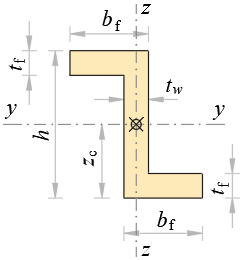### Geometrical Properties of Zed SectionDimensions

h = m, tw = m

bf = m, tf = m

Area

Aw = h·tw m2

Af = (bftwtf m2

A = Aw + 2·Af m2

Centroid

yc = bftw2 m

zc = h2 m

Perimeter

P = 2·(h + 2·bftw) m

Second moments of area

Iy = bf·h3 – (bftw)·(h – 2·tf)312 m4

Iz = (h – 2·tftw3 + 2·tf·bf·(bf2 + 3·(bftw)2)12 m4

Iyz = -bf·(htfAf2 m4

Principal moments of area

I1 = Iy + Iz2 +  (IyIz)24 + Iyz2 m4

I2 = Iy + Iz2 –  (IyIz)24 + Iyz2 m4

Angle of principal axis

α1 = atan(IyI1Iyz) о

Polar moment of area

Ix = I1 + I2 m4